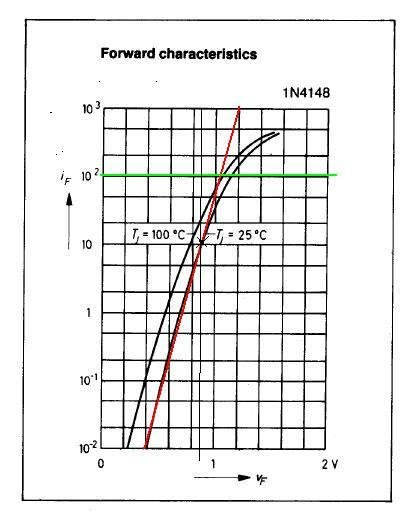# SPICE modeling of a Diode from Datasheet

The M coefficient is not in the datasheet, it’s a value that ranges between 0.25 and 0.45 for most of the diodes. For low voltage Zener diode we assume M = 0.25, for diodes that work on voltages from 50 to 100V M = 0.33 and for several hundred volts we can assume M = 0.45. Schottky diodes have a coefficient M, which typically varies from 0.4 to 0.5. In this case M = 0.33.

The transit time TT for switching diodes of this type is a value close to 10ns. In the case of ultrafast diodes rectifiers is up to 50ns, while for a fast rectifiers up to 250ns.For Zener diodes, with voltages below 10V TT is around 200ns, while for voltages exceeding 100V is up to 3ms. In our case TT = 10ns.

For EG we can assume the standard value of 1.1 eV, while it’s 0.7 eV for Schottky diode and 0.67 eV for germanium diodes.

The N value ranges between 1.0 and about 2.0.Assume N = 2.0.For determining IS and RS, we need the following forward I-V characteristic curve that we derive from the datasheet:Let’s trace the line that goes to about half of the linear curve that intersects the value of current ID to 10mA, which corresponds to a value of about VD 0.84V.Because must be:

ID = IS*(e^(VD/(N*Vt)) -1)

with N = 2.0 and Vt = 0.026V, IS is about 1nA.We can estimate RS as voltage difference on the green line drawn at the point of the knee of the curve, and the red line, divided by the corresponding current, hence:

RS =0.1V/0.1A = 1 Ohm

Considering as characteristic values of VJ = 0.8V for these diodes, CF = 0.5 and IKF that can be taken as equal to the average rectified current Io 150mA, our first model could be:

.model D1N4148 D(Is=1n N=2 Rs=1 Ikf=150m Xti=3 Eg=1.11 Cjo=4p

+ M=.3333 Vj=.8 Fc=.5 Bv=100 Ibv=100u Tt=10n)

The omitted parameters are taken with the default values. At this point we can open the “PSpice Model Editor” and click on “New” from menu “File” and then “New” from menu “Model”:Name the model and click on OK:Posted in PSpice Modeling from Datasheet and tagged , , , .